# Standard Atmospheric Refraction: Empirical Evidence and Derivation

#### Everett Anderson

##### New Member
1) How we know standard atmospheric refraction is a real phenomena and
2) Why you used a value of 7/6*R for it [in the Metabunk curve calculator]

Last edited by a moderator:

#### Mick West

Staff member
Atmospheric refraction is measurable when observing stars. Stars visually appear to rotate around the Earth once ever sidereal day - and they do this at a constant angular speed. However as they approach the horizon they seem to slow down a little, basically staying visible longer than they should. When they reach the horizon their actual calculated position is a bit over 0.5° below the horizon - a bit more than the diameter of the sun.

This amount matches what we have empirically found about the refractive index of air at various temperatures and pressures, and what we have empirically found about the change in temperature and pressure at various altitudes in the atmosphere, and what we have empirically determined to be the radius of the Earth.

The refraction of terrestrial objects differs from stars in that only part of the atmosphere, whereas astronomical observation passes through the entire atmosphere.

The value of 7/6*R is just a rough approximation of the effect of refraction on the perceived curvature of the Earth. The actual radius, R, is 3959 miles, but a standard atmosphere bends light down slightly making it look like the earth curves less, so we use 7/6*R as an apparent radius (i.e roughly matching what we see)." It's very approximate as the atmosphere is quite variable. Basically though you do the same calculations, but the larger radius gives you the reduced curvature.

I initially got the 7/6 value from:
http://aty.sdsu.edu/explain/atmos_refr/horizon.html

If we can assume a constant lapse rate in the air between the eye and the Earth's surface, and if the observer's height h is small compared to the 8-km height of the homogeneous atmosphere, then we can assume the curved ray is an arc of a circle. This assumption makes things easy, because the relative curvature of the ray and the Earth's surface is all that matters. In effect, we can use the previous result, but just use an effective radius of curvature for the Earth that is bigger than the real one.

This assumption is made so often that it's conventional in surveying and geodesy to use a “refraction constant” that's just the ratio of the two curvatures. A typical value of the ratio is about 1/7; that is, the ray curves about 1/7 as much as the Earth does (or, equivalently, the radius of curvature of the ray is about 7 times that of the Earth's surface).

Using this “typical” value means we should just use the formula given above, but use a value R′ instead of R for the effective radius of the Earth, where

1/R′ = 1/R − 1/(7R) = 6/(7R) ,
so that
R′ = R × 7/6 .
Content from External Source
The main ways in which observations will differ from the standard refraction are that the temperature near the ground often varies greatly from a few meters above. Also the lapse rate (how quickly the air cools) may not be linear, especially at low altitudes. This can result in vertical stretching and/or compression of the image.

If you want more details, including empirical observations, I recommend this set of tests by the USGS:
https://www.ngs.noaa.gov/PUBS_LIB/ResultsOfLevelingRefractionTests_by_NGS_TR_NOS92_NGS22.pdf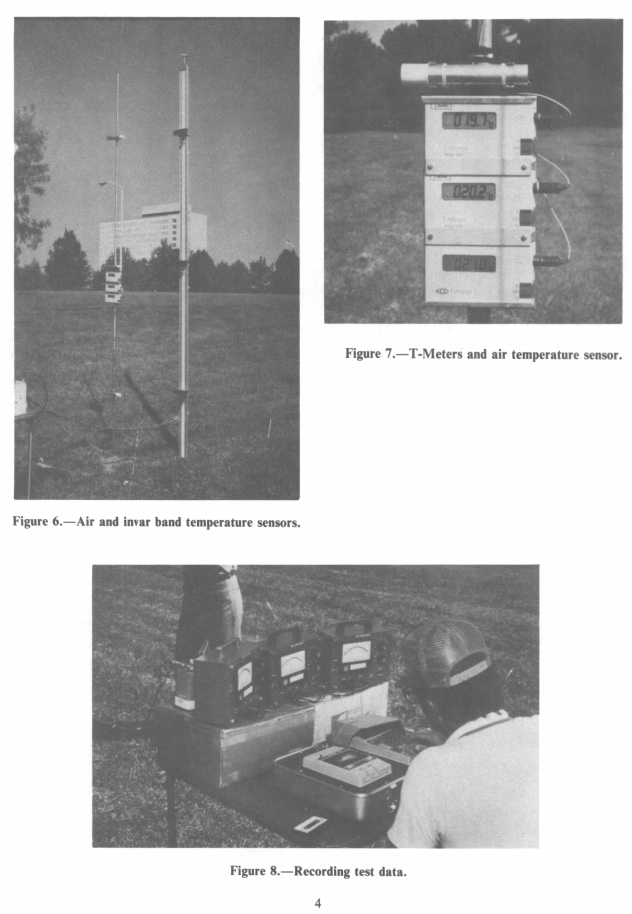Last edited:

Staff member

#### OTACHI

##### New Member
Does it always happen?

#### Mick West

Staff member
Does it always happen?
There's always some refraction yes, because of the pressure gradient of the atmosphere. The actual amount and nature of this can vary - like if there's a temperature inversion.
https://en.wikipedia.org/wiki/Horizon#Effect_of_atmospheric_refraction
If the Earth were an airless world like the Moon, the above calculations would be accurate. However, Earth has an atmosphere of air, whose density and refractive index vary considerably depending on the temperature and pressure. This makes the air refract light to varying extents, affecting the appearance of the horizon. Usually, the density of the air just above the surface of the Earth is greater than its density at greater altitudes. This makes its refractive index greater near the surface than higher, which causes light that is travelling roughly horizontally to be refracted downward. This makes the actual distance to the horizon greater than the distance calculated with geometrical formulas. With standard atmospheric conditions, the difference is about 8%. This changes the factor of 3.57, in the metric formulas used above, to about 3.86. This correction can be, and often is, applied as a fairly good approximation when conditions are close to standard. When conditions are unusual, this approximation fails. Refraction is strongly affected by temperature gradients, which can vary considerably from day to day, especially over water. In extreme cases, usually in springtime, when warm air overlies cold water, refraction can allow light to follow the Earth's surface for hundreds of kilometres. Opposite conditions occur, for example, in deserts, where the surface is very hot, so hot, low-density air is below cooler air. This causes light to be refracted upward, causing mirage effects that make the concept of the horizon somewhat meaningless. Calculated values for the effects of refraction under unusual conditions are therefore only approximate.[5
Content from External Source

#### Clouds Givemethewillies

##### Senior Member
"Please be aware that the preceding argument contains several unrealistic assumptions. For example, the lapse rate near the ground is never constant, so the path of the horizon ray is never an arc of a circle. Fortunately, in calculating the dip of the horizon, this idealization makes very little difference. In our paper on the dip diagram, George Kattawar and I showed that the dip depends almost entirely on the difference in temperature between the air at eye level and that at the apparent horizon, regardless of the thermal structure in between. "
Content from External Source
http://aty.sdsu.edu/explain/atmos_refr/dip.html

Last edited by a moderator:

#### Mick West

Staff member
I made a video analyzing the relative slowdown of stars as they get closer to the horizon:

Summed up in this image: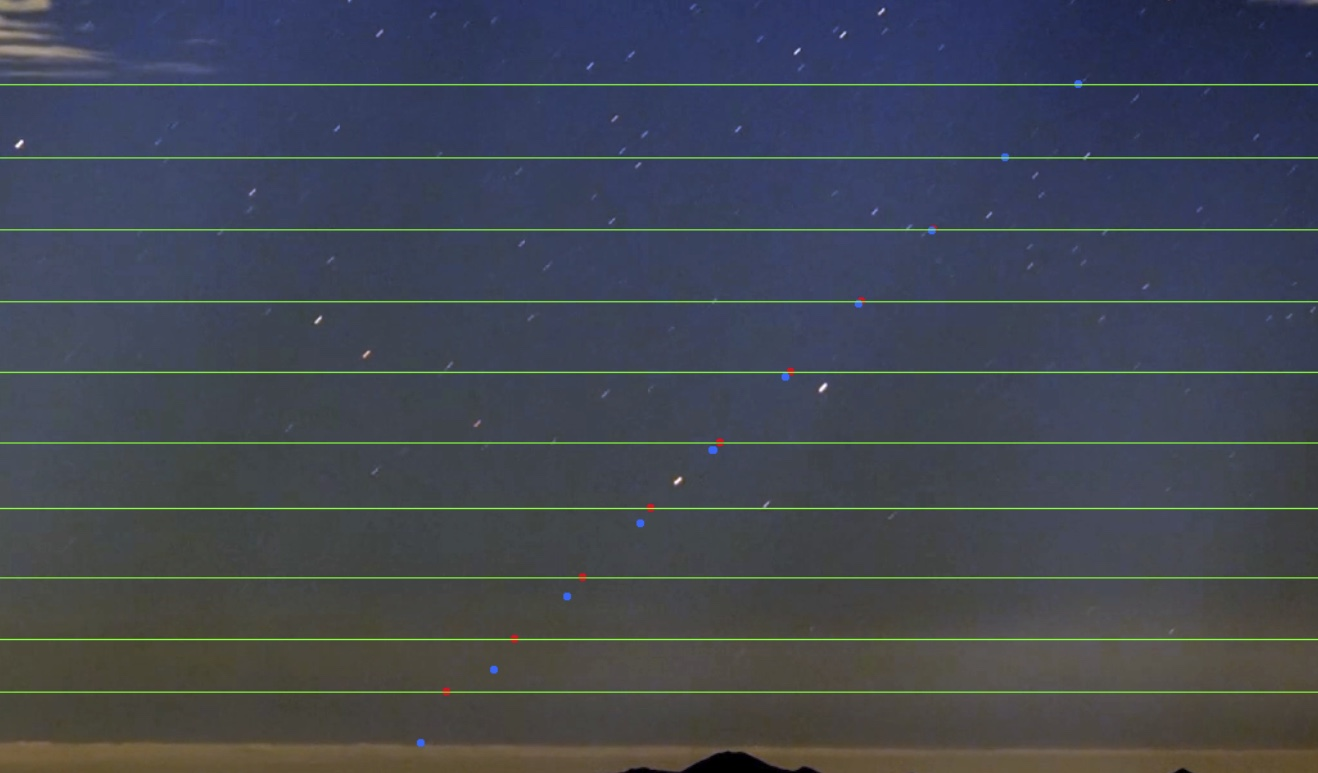What I did was track one star and put a red dot (and green horizontal line) where it was every one second.

I then took the first two positions and used them to extrapolate expected positions at a constant speed.

The results show that the star is already slowing down a bit, and the slowdown rapid increases as you get closer to the horizon.

#### Mick West

Staff member
Looking for more derivations of the 7/6 (1.1666) value.

https://en.wikipedia.org/wiki/Atmospheric_refraction

As a common approximation, terrestrial refraction is considered as a constant bending of the ray of light or line of sight, in which the ray can be considered as describing a circular path. A common measure of refraction is the coefficient of refraction. Unfortunately there are two different definitions of this coefficient. One is the ratio of the radius of the Earth to the radius of the line of sight, the other is the ratio of the angle that the line of sight subtends at the center of the Earth to the angle of refraction measured at the observer. Since the latter definition only measures the bending of the ray at one end of the line of sight, it is one half the value of the former definition.

The coefficient of refraction is directly related to the local vertical temperature gradient and the atmospheric temperature and pressure. The larger version of the coefficient k, measuring the ratio of the radius of the Earth to the radius of the line of sight, is given by: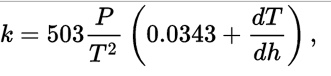https://wikimedia.org/api/rest_v1/media/math/render/svg/e4c90d6e96eacccaf3740d74df75ed4d605fc14f
where temperature T is given in degrees Kelvin, pressure P in millibars, and height h in meters. The angle of refraction increases with the coefficient of refraction and with the length of the line of sight.

Although the straight line from your eye to a distant mountain might be blocked by a closer hill, the ray may curve enough to make the distant peak visible. A convenient method to analyze the effect of refraction on visibility is to consider a reduced effective radius of the Earth Reff, given by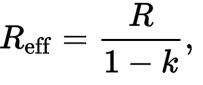https://wikimedia.org/api/rest_v1/media/math/render/svg/7a13c6fb5428cd6492d17f8182635c0c4465185f
where R is the radius of the Earth and k is the coefficient of refraction. Under this model the ray can be considered a straight line on an Earth of increased radius.
Content from External Source
For a standard atmosphere at mean sea level, P= 1013.25, T=288.15°K (15°C), dT/dH = 6.5/1000.
Hence, k = 503*1013.25/288.15^2*(0.0343 + 6.5/1000) = 0.25, so Reff = 1.333*R, as opposed to 1.166R

Is the seconds equation supposed to use the "smaller" k? Did I make a mistake? Reference 11 is attached, in which Andy Young says: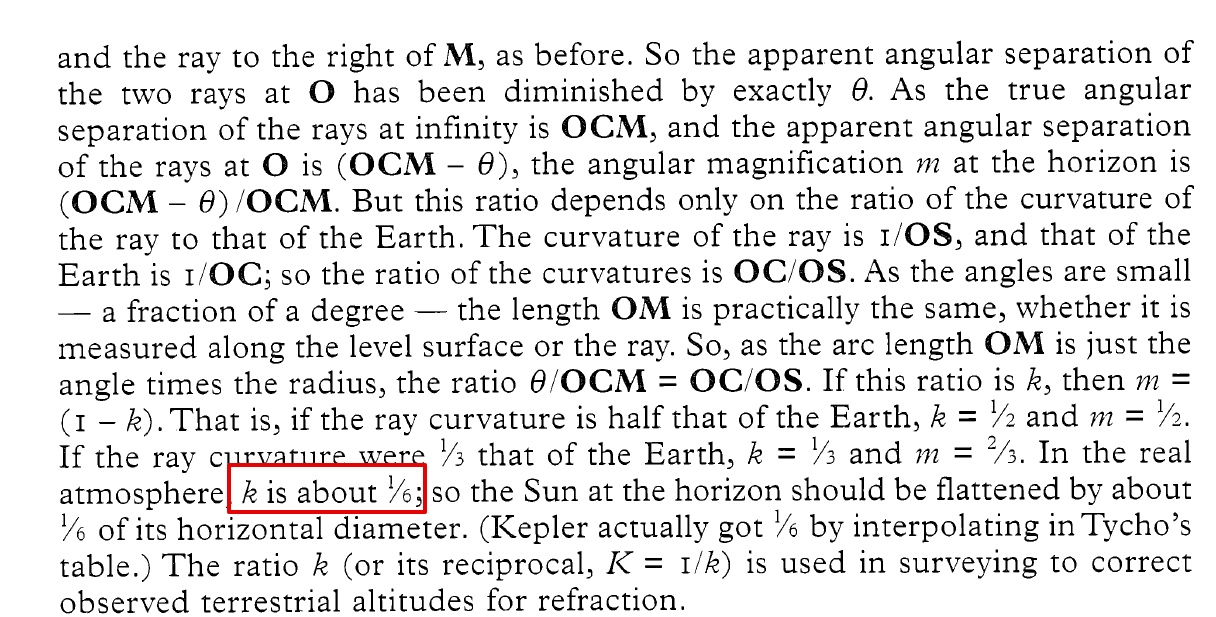1/6 = 0.16666, giving Reff of 1/(1-1/6)*R = 1.2*R.

The 7/6 is just a ballpark, but it would be good to to verify the derivation of this number (and clarify which "k" is used. It's probably in Dr. Young's document.

#### Attachments

• 2006Obs___126___82Y-OCR.pdf
15.6 MB · Views: 2,123
Last edited:

#### Mick West

Staff member
Different people use different numbers. I wonder if the calculator should show a range. Maybe even generate a diagram of horizon lines.

Atmospheric Refraction: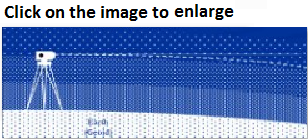Refraction is largely a function of atmospheric pressure and temperature gradients, which may cause:

the bending to be up or down by extremely variable amounts.

There are basically three types of temperature gradient (dT/dh):

1. Absorption: occurs mainly at night when the colder ground absorbs heat from the atmosphere.

• This causes the atmospheric temperature to increase with distance from the ground and dT/dh > 0.
2. Emission: occurs mainly during the day when the warmer ground emits heat into the atmosphere, resulting in a negative temperature gradient, i.e. dT/dh < 0.

3. Equilibrium: no heat transfer takes place (dT/dh = 0) and occurs only briefly in the evening and morning.

4. The result of dT/dh < 0 is to cause the light ray to be convex to the ground rather than concave as generally shown.
• This effect increases the closer to the ground the light ray gets and errors in the region of 5 mm/km have resulted.
The atmosphere refracts the horizontal line of sight downward, making the level rod reading smaller. The typical effect of refraction is equal to about 14% of the effect of earth curvature.
Content from External Source
So they say "14% of the effect of earth curvature.", is this the same as using a radius of 1.14*R? Not really clear what they mean there.

#### Clouds Givemethewillies

##### Senior Member
The result of dT/dh < 0 is to cause the light ray to be convex to the ground rather than concave as generally shown.

I think they got that wrong for a start. Even if the temperature is constant with height the density will drop due to pressure drop.

A typical lapse rate, which is limited to the dry adiabatic rate, would decrease refraction somewhat.

Last edited:

#### Mick West

Staff member
I think they got that wrong for a start. Even if the temperature is constant with height the density will drop due to pressure drop.

A typical lapse rate, which is limited to the dry adiabatic rate, would decrease refraction somewhat.

But if you've got a warm region lower down the expansion of the air due to heating reduces the density, cause the bend upwards. Hence some types of mirages.

#### Clouds Givemethewillies

##### Senior Member
But if you've got a warm region lower down the expansion of the air due to heating reduces the density, cause the bend upwards. Hence some types of mirages.
But the air is unstable, and convection and mixing generally occurs. A hot road is slightly different in that inversion can be stable in a very thin layer, the thermal boundary layer, I think.

#### Hamster99

##### New Member
Different people use different numbers. I wonder if the calculator should show a range. Maybe even generate a diagram of horizon lines.

It might be nice if the calculator had a range of values indicating extreme refraction but I have used a method of changing the value of Radius in my own calculator. My problem has been in determining if that value is achievable in real life.
Your calculator is very valuable for evaluating flat earth claims

Last edited by a moderator:

#### Mick West

Staff member
It might be nice if the calculator had a range of values indicating extreme refraction but I have used a method of changing the value of Radius in my own calculator. My problem has been in determining if that value is achievable in real life.
Your calculator is very valuable for evaluating flat earth claims

The problem with a range is that the more extreme examples of refraction are not at all linear. i.e. you can't simply say there's 1/6 or more visible. What often happens is that the view is over cool water which mostly bends the light paths near the horizon down over the curve and creates a highly compressed image of behind-the-horizon objects. So you can't really reduce that to a simple number.

Last edited:

#### Clouds Givemethewillies

##### Senior Member
The problem with a range is that the more extreme examples of refraction are not at all linear. i.e. you can't simply say there's 1/6 or more visible. What often happens is that the view is over cool water which mostly bends the light paths near the horizon down over the curve and creates a highly compressed image of behind-the-horizon objects. So you can't really reduce that to a simple number.

The effect of refraction looking at the tops of mountains (even small ones!) should be more predictable than objects closer to sea level, as the lapse rate will tend to average out over a greater height difference between object and observer. If the top of a mountain pokes through the top of the planetary boundary layer (~1-2km.} there is often an inversion there, which might possibly increase the range at which at which the tops of mountains can be seen.

#### Hamster99

##### New Member
The problem with a range is that the more extreme examples of refraction are not at all linear. i.e. you can't simply say there's 1/6 or more visible. What often happens is that the view is over cool water which mostly bends the light paths near the horizon down over the curve and creates a highly compressed image of behind-the-horizon objects. So you can't really reduce that to a simple number.
True but annoying.#### Michael Onines

##### New Member
Looking for more derivations of the 7/6 (1.1666) value.

https://en.wikipedia.org/wiki/Atmospheric_refractionhttps://wikimedia.org/api/rest_v1/media/math/render/svg/e4c90d6e96eacccaf3740d74df75ed4d605fc14f
where temperature T is given in degrees Kelvin, pressure P in millibars, and height h in meters. The angle of refraction increases with the coefficient of refraction and with the length of the line of sight.

Content from External Source

The equation cited is from Hirt, et al, 2010, and appears to have been empirically derived from a long series of simultaneous reciprocal observations and back-calculating the refraction component from those observations. They then built an empirical relationship between the temperature gradient and the refraction correction. I haven't read their paper, but the technique of calculating refraction corrections from simultaneous reciprocal observation appears to have the earth's radius as one of its assumptions, so FE doing a deep dive into it will find a somewhat circular reasoning.

One reason you are getting much higher k value than is normally used is you plugged in a temperature inversion when you used a positive value for dT/dh. In "standard conditions" the temperature drops as you get higher, so dT/dh is negative. Using dT/dh = -0.0065 gets to within about 3% of the 1/6 of curvature drop rule of thumb for standard refraction. If you use a very strong negative gradient, the value of k goes negative, which is what you see with a road "mirroring" the sky on a hot sunny day.

A derivation from basic principles of a similar refraction correction equation (with an added humidity gradient correction) is found in Torge's "Geodesy". In the 2nd edition (1991) the derivation is in section 4.3.1, and the entire section is in Google Books' preview of the book. In the 3rd edition it is section 5.1, 5.1.1, and 5.1.2, but in Google Books' preview two pages of these sections are omitted, so you will have to look for those pages in a library. The two derivations differ slightly in presentation, and the third edition relies on a new set of atmospheric index of refraction equations adopted by the International Association of Geodesy in 1999, but the end result is the same, as several of the coefficients have been rounded and the newer index of refraction model was in general agreement with the previously used equations. Torge's derivation ends with equation 4.40 (2nd ed) or 5.19a (3rd ed), which doesn't quite give it in a form where k is calculated directly. Baselga 2014 takes that and plugs it into one of the preceding equations in Torge's derivation to present

Baselga et al said:
k = (R / 10^6) * {78 * (p / T^2) *(0.034+dT/dz ) + (11 / T) * de/dz }

The Baselga paper is a good background document that describes the different approximations and techniques surveyors have used for atmospheric refraction correction. "Practical Formulas for the Refraction Coefficient" 2014 by Baselga et. al. This paper also has an example of how significantly refraction corrections can vary with different atmospheric conditions, even at a single site over the course of 24 hours.

Last edited:

#### ImRic

##### New Member
I just signed up to make a suggestion on your curve calculation page.
The diagram below does not show the level line that would measure the drop in your chart. I hope you will consider adding the drop line as this is a major hurdle in explaining drop vs bulge to flat earthers.

#### Rory

##### Closed Account
1) How do we know atmospheric refraction is a real phenomenon?
Here's an interesting home demonstration someone did, where they created a layer of cool air which brought into view something that was hidden by a horizon: